# Flux Calibration Example Using HST image¶

From an HST image contained in our field of view, we compute the calibration bias of our SITELLE data cube.

We integrate our sitelle data in the HST filter bandpass. The obtained ‘flux map’ is compared pixel by pixel to the HST flux map to compute the flux bias

:

#Should be removed in a regular python script
%matplotlib notebook
from astropy import wcs
from orcs.process import SpectralCube
import numpy as np
from astropy.io import fits
import matplotlib.pyplot as plt
from scipy.interpolate import RectBivariateSpline
from orcs.core import Filter
from orb.utils.image import nanbin_image

/home/blaunet/sitelle_env/lib/python2.7/site-packages/h5py/__init__.py:36: FutureWarning: Conversion of the second argument of issubdtype from float to np.floating is deprecated. In future, it will be treated as np.float64 == np.dtype(float).type.
from ._conv import register_converters as _register_converters


We load the SITELLE data and adjust the WCS calibration (done beforehand)

:

SN2 = SpectralCube('/data/blaunet/fits/orig/M31_SN2.merged.cm1.1.0.hdf5')
SN2.set_wcs('./calibrations_maps/direct.wcs.deep_frame.fits')
SN2.set_dxdymaps('./calibrations_maps/direct.wcs.dxmap.fits', './calibrations_maps/direct.wcs.dymap.fits')

INFO| Data shape : (2048, 2064, 556)
WARNING| /home/blaunet/orcs/orcs/core.py:1586: UserWarning: Malformed spectral cube. The number of steps in the header (900) does not correspond to the real size of the data cube (556)
warnings.warn('Malformed spectral cube. The number of steps in the header ({}) does not correspond to the real size of the data cube ({})'.format(step_nb, self.dimz))

INFO| Cube is in WAVENUMBER (cm-1)
INFO| Cube is CALIBRATED in wavenumber
WARNING| /home/blaunet/orb/orb/core.py:416: UserWarning: Parameter already defined

INFO| Cube is in WAVENUMBER (cm-1)
INFO| Cube is CALIBRATED in wavenumber
WARNING| /home/blaunet/orcs/orcs/core.py:1441: UserWarning: dxmap reshaped from (200, 200) to (2048, 2064)
format(dxmap.shape, self.dimx, self.dimy))

WARNING| /home/blaunet/orcs/orcs/core.py:1448: UserWarning: dymap reshaped from (200, 200) to (2048, 2064)
format(dymap.shape, self.dimx, self.dimy))



We load our HST data. We are interested in the extension n°1 of the fits file

:

hst_file_path = '/data/blaunet/fits/HLA/hst_11714_96_wfc3_uvis_f502n/hst_11714_96_wfc3_uvis_f502n_drz.fits'
xmin, xmax = 980, 1170
ymin, ymax = 940, 1130

ext_num = 1

:

hst_data = fits.getdata(hst_file_path, ext_num).T #Transpose to match ORCS convention on indexing [x,y]
hst_data = hst_data*PHOTFLAM # We want our data to be in erg/cm2/A/s
hst_wcs = wcs.WCS(hst_header) #We need the WCS to make the two data set correspond

:

f,ax = plt.subplots()
ax.imshow(hst_data.T, origin='lower', cmap='gray_r',
vmin = np.nanpercentile(hst_data, 5),
vmax = np.nanpercentile(hst_data, 95))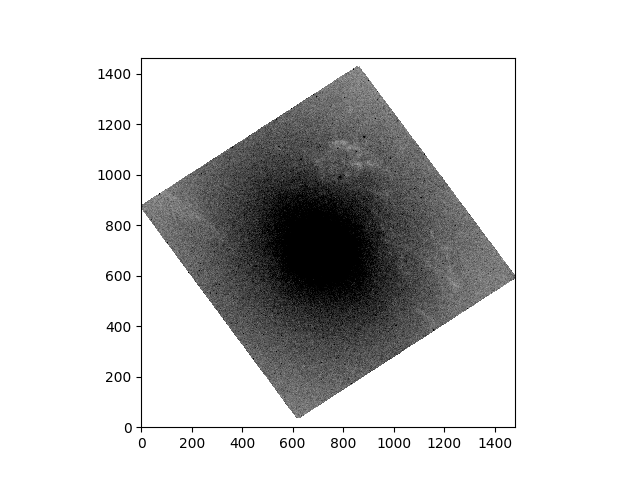:

<matplotlib.image.AxesImage at 0x7f724e261390>


## Instanciation of a Filter¶

We need to get the filter corresponding to our HST image

:

hst_filter_path = '/data/blaunet/fits/HST/HST_WFC3_UVIS1.F502N.dat.txt'

:

a = []
T = []
with open(hst_filter_path, 'r') as f:
for line in f:
tmp = map(float,line.strip().split(' ')) # We convert the line elemtnts into floats
a.append(tmp/10.) # to have nm
T.append(tmp)
filter_axis = np.array(a) #the list are converted to numpy array for convenience
filter_transmission = np.array(T)
hst_filter = Filter(filter_axis, filter_transmission) # a Filter object is istanciated


### Integration of SITELLE data in HST filter bandpass¶

For speed concerns, we don’t need to perform this integration over the whole SITELLE field of view, as HST images are usually much smaller. From image study, we determined boundaries of HST image in our field of view

:

#Outside this region, the flux is set to NaN
sitelle_flux_map = SN2.integrate(hst_filter, xmin,xmax,ymin,ymax)
sitelle_flux_map[sitelle_flux_map == 0.] = np.nan

:

f,ax = plt.subplots()
im = ax.imshow(sitelle_flux_map.T, origin='lower', cmap='RdBu_r')
ax.set_xlim(xmin, xmax)
ax.set_ylim(ymin,ymax)
f.colorbar(im)
ax.set_title('Integrated SITELLE flux in HST F502N filter')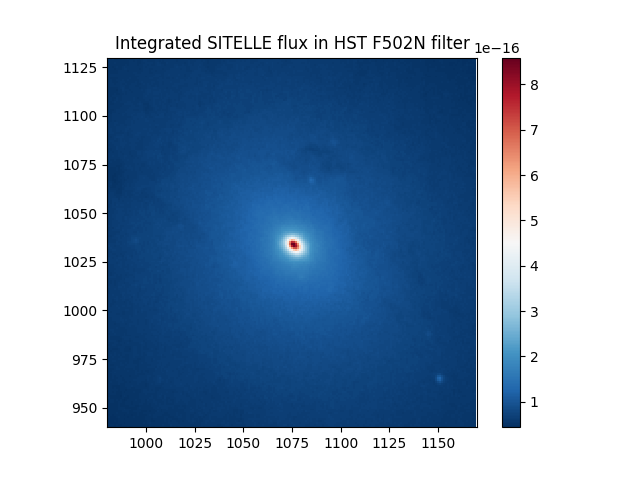:

Text(0.5,1,u'Integrated SITELLE flux in HST F502N filter')


### Reprojection of HST data in SITELLE coordinates¶

We are going to interpolate HST data on our SITELLE grid. Because the pixel size are obviously not the same, we need to be careful in how we do that.

astropy WCS objects provide useful methods to do this (see http://docs.astropy.org/en/stable/api/astropy.wcs.utils.proj_plane_pixel_area.html#astropy.wcs.utils.proj_plane_pixel_area)

:

print 'SITELLE pixel area in the "projection plane" : ', wcs.utils.proj_plane_pixel_area(SN2.get_wcs())
print 'HST pixel area in the "projection plane" : ', wcs.utils.proj_plane_pixel_area(hst_wcs)

pixel_scale_factor = wcs.utils.proj_plane_pixel_area(SN2.get_wcs())/wcs.utils.proj_plane_pixel_area(hst_wcs)
print 'Pixel scale factor (SITELLE/HST) : ', pixel_scale_factor

SITELLE pixel area in the "projection plane" :  8.092914404881915e-09
HST pixel area in the "projection plane" :  1.1926811840401893e-10
Pixel scale factor (SITELLE/HST) :  67.85480070597988


Therefore, we are going to intentionally oversample our HST image, bin it and correct by the pixel sacle factor

:

scale_factor = np.ceil(np.sqrt(pixel_scale_factor))
X,Y = np.mgrid[xmin:xmax:1/scale_factor,ymin:ymax:1/scale_factor]  #This is an ovsersampled grid
XYp = hst_wcs.all_world2pix(SN2.pix2world(np.array([X.flatten(),Y.flatten()]).T), 0)
Xp, Yp = XYp.T


Xp, Yp are now the coordinates of an oversampled SITELLE grid in HST image coordinates.

We perform a linear interpolation of HST image on this grid

:

# If we have NaNs it's going to mess with the interpolation
hst_data = fits.getdata(hst_file_path, ext_num).T*PHOTFLAM
nan_pos = np.isnan(hst_data)
hst_data[nan_pos] = 0.

:

projection_function = RectBivariateSpline(np.arange(hst_data.shape),
np.arange(hst_data.shape),
hst_data, kx=1, ky=1, s=0)
reprojected_HST = projection_function.ev(Xp.reshape(*X.shape),Yp.reshape(*Y.shape))


reprojected_HST is now HST image evaluated on the oversampled SITELLE grid.

We now bin this to SITELLE’s resolution. As nanbin_image computes the binned mean, we have to correct for the pixel area

:

hst_flux_map = np.full_like(sitelle_flux_map, np.nan)
hst_flux_map[xmin:xmax, ymin:ymax] = nanbin_image(reprojected_HST, int(scale_factor))*pixel_scale_factor
hst_flux_map[hst_flux_map == 0.] = np.nan


We plot the resulting map

:

f,ax = plt.subplots()
im = ax.imshow(hst_flux_map.T, origin='bottom-left', cmap='jet')
ax.set_xlim(xmin, xmax)
ax.set_ylim(ymin,ymax)
f.colorbar(im)
ax.set_title('HST Flux map, in SITELLE grid')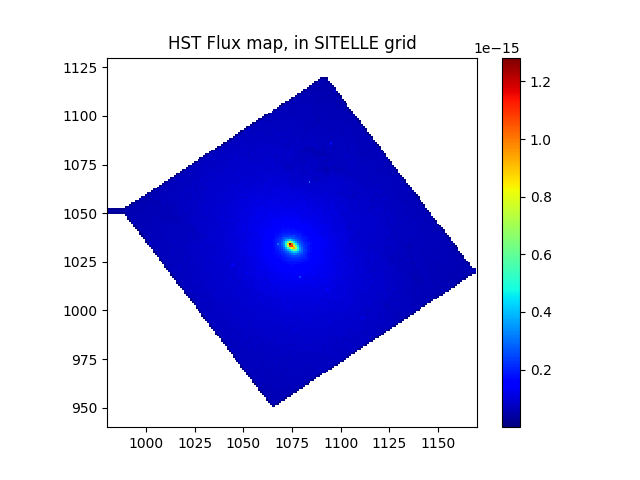:

Text(0.5,1,u'HST Flux map, in SITELLE grid')


### Flux Ratio¶

From this two maps, we can get the flux ratio (i.e. bias) between our datacube and HST data

:

ratio = hst_flux_map/sitelle_flux_map
f,ax = plt.subplots()
im = ax.imshow(ratio.T, origin='bottom-left', cmap='jet',
vmax = np.nanpercentile(ratio, 99),
vmin=np.nanpercentile(ratio,1))
ax.set_xlim(xmin, xmax)
ax.set_ylim(ymin,ymax)
f.colorbar(im)
ax.set_title('Ratio of HST flux map over SITELLE flux map')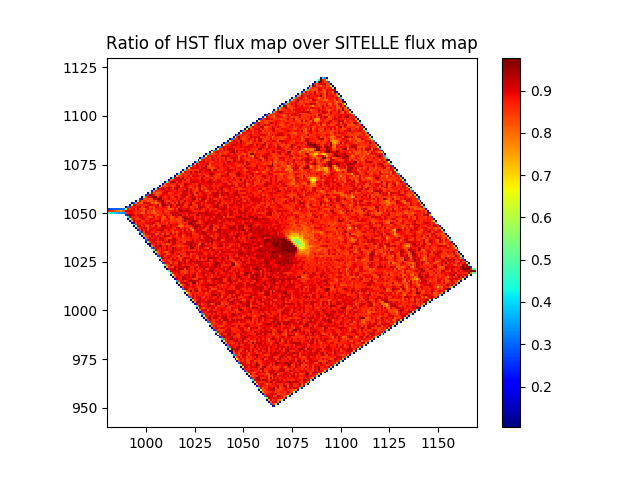:

Text(0.5,1,u'Ratio of HST flux map over SITELLE flux map')


This can also be plotted as an histogram

:

f,ax = plt.subplots()
h = ax.hist(ratio[~np.isnan(ratio)], bins=300) # we need to remove the NaNs
ax.set_title('Median = %.5f, Std = %.5f'%(np.median(ratio[~np.isnan(ratio)]), np.std(ratio[~np.isnan(ratio)])))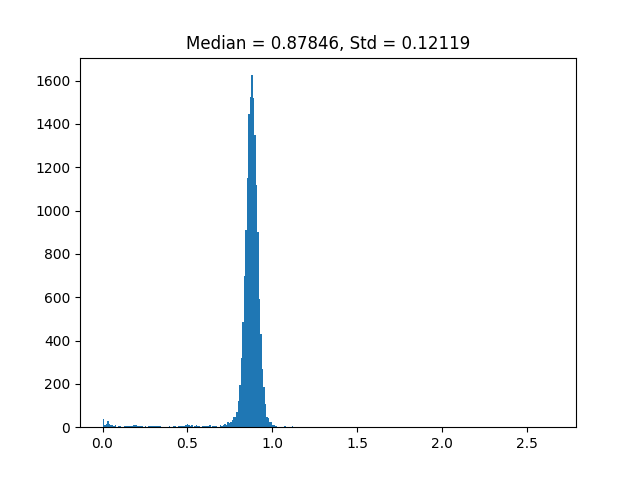:

Text(0.5,1,u'Median = 0.87846, Std = 0.12119')

[ ]:




### The End¶

From now on, it’s up to the user to choose what to do with this : use it as is or try to clean this map/histogram from obvious outliers (edge effects, center of the frame).

What follows may thus be applicable only to this specific data, and be conceptually arguable

:

#We consider extrema at 1% and 99% as outliers
r = np.copy(ratio)
r[r > np.nanpercentile(ratio,99)] = np.nan
r[r < np.nanpercentile(ratio,1)] = np.nan

WARNING| /home/blaunet/sitelle_env/lib/python2.7/site-packages/ipykernel_launcher.py:3: RuntimeWarning: invalid value encountered in greater
This is separate from the ipykernel package so we can avoid doing imports until

WARNING| /home/blaunet/sitelle_env/lib/python2.7/site-packages/ipykernel_launcher.py:4: RuntimeWarning: invalid value encountered in less
after removing the cwd from sys.path.


:

f,ax = plt.subplots()
ax.hist(r[~np.isnan(r)], bins=300) # we need to remove the NaNs
ax.set_title('Median = %.5f, Std = %.5f'%(np.median(r[~np.isnan(r)]), np.std(r[~np.isnan(r)])))

f,ax = plt.subplots()
im = ax.imshow(r.T, origin='lower', cmap='jet')
ax.set_xlim(xmin, xmax)
ax.set_ylim(ymin,ymax)
plt.colorbar(im)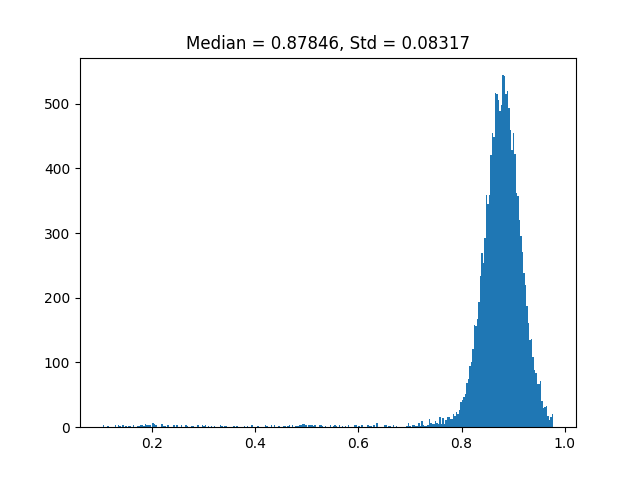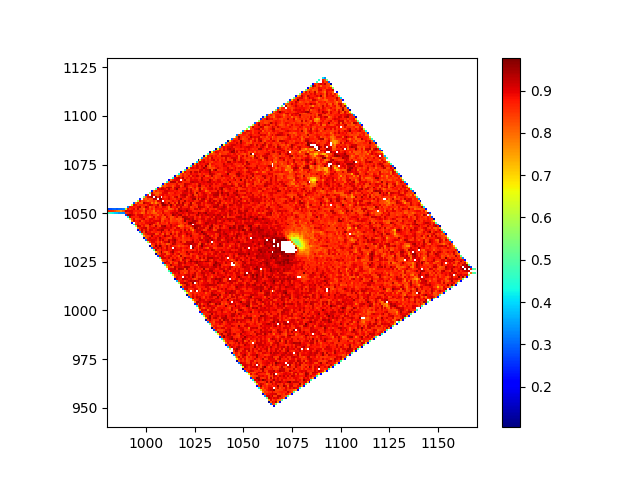:

<matplotlib.colorbar.Colorbar at 0x7f7247736d50>

[ ]:



[ ]: